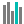#DEVSQ (PQL - xl)

This function calculates the sum of the squares of deviations of data points (or of an array of data points) from their sample mean

• Library: PQL \ Spreadsheet \ Statistical
• Compatibility: Any content (regardless of data source) in the Tabulate spreadsheet module

#### Syntax

DEVSQ(number1, number2)

##### Function Arguments
 Name Description Type Optional number1 Double-precision floating-point value, an integer value, or an array (cell range) of these StringOrNumber number2 Double-precision floating-point value, an integer value, or an array (cell range) of these StringOrNumber